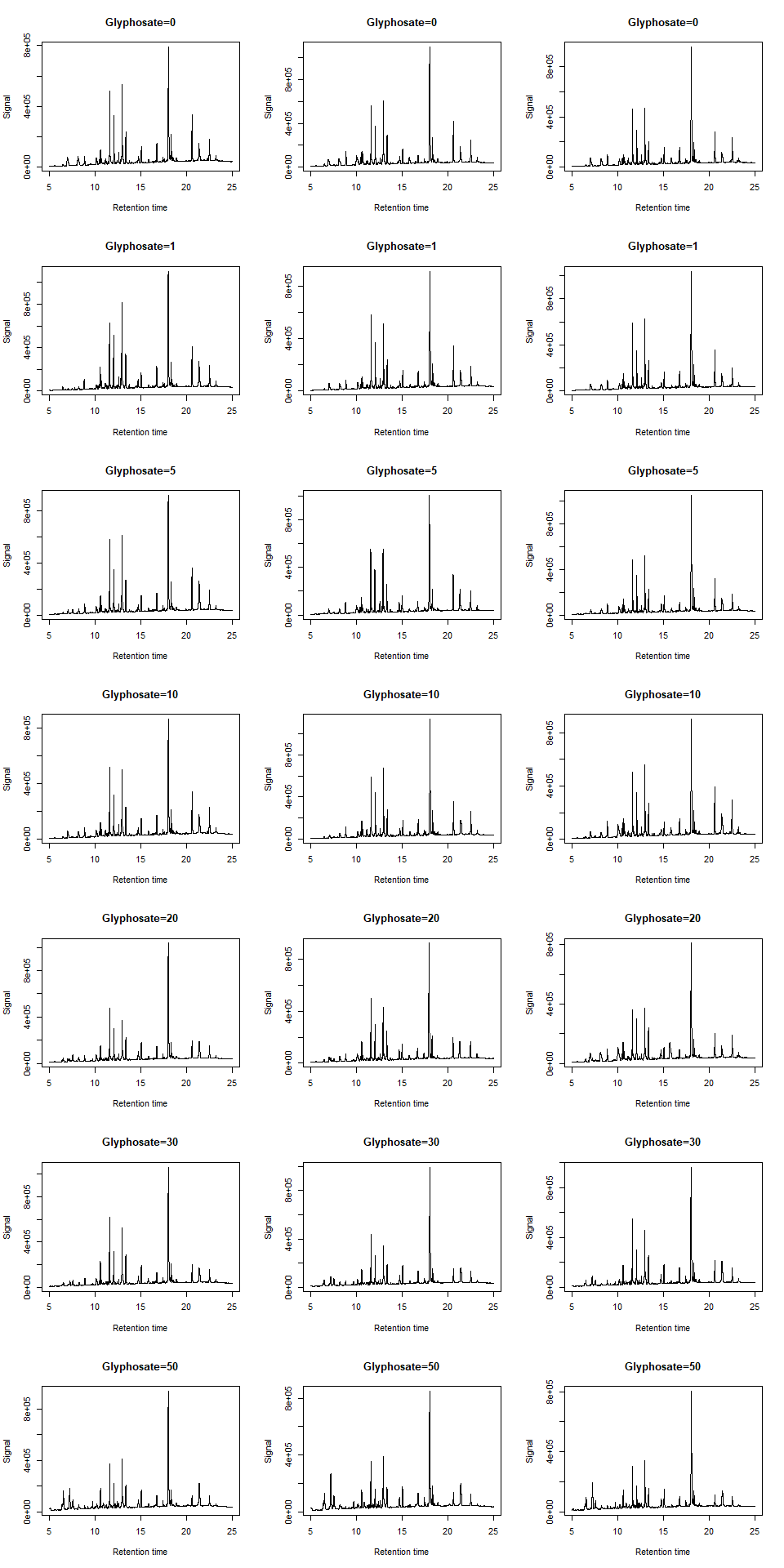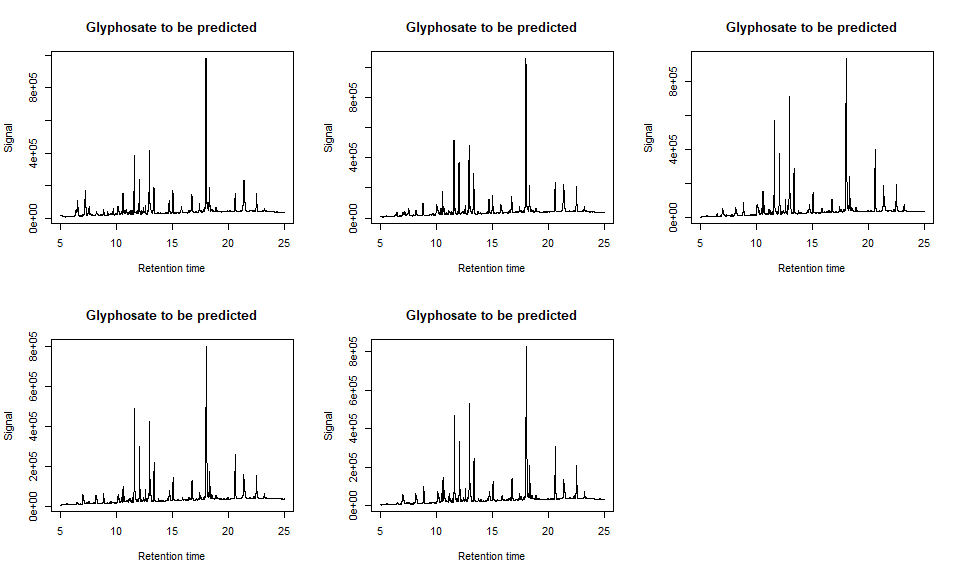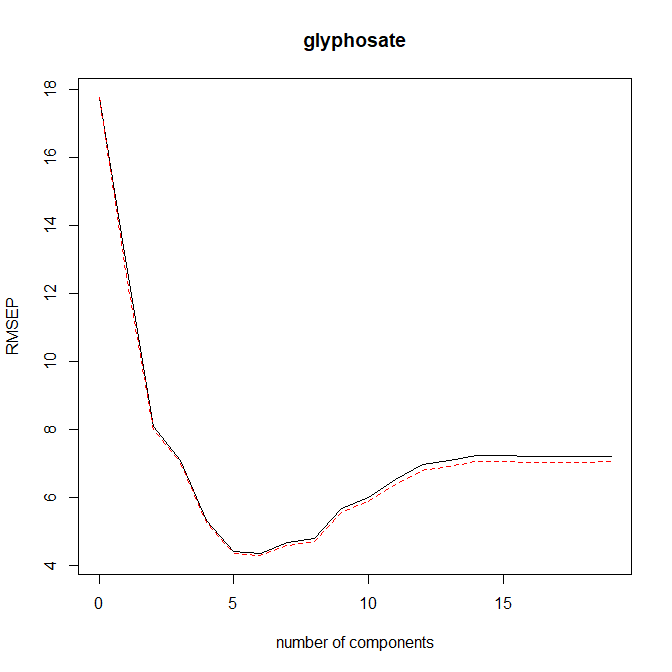# Chemometrics with Partial Least Squares Regression and Principal Component Regression in R

Potentially, plants can be used as indicators (biomarkers) of soil and water pollution. Suitably informative measurements on the plants may indicate presence of certain chemicals. The present data contain measurements on 26 plants exposed to different amounts of glyphosate, which is the active component of certain herbicides. The plants are measured by UHPLC-DAD (Ultra-High-Performance Liquid Chromatography-Diode-Array- Detection) which results in a chromatogram for each plant. This chromatogram contains 24000 readings of intensities for 24000 corresponding retention times. Thus, for each wavelength we basically have observed intensity as function of retention time. A peak at a certain retention time is supposed to represent a high quantity of a specific chemical component. Retention times may “slide” a little from analysis to analysis. (In fact, by this measuring technique, many chromatograms, corresponding to many wavelengths, are observed for each plant, but we only use a single wavelength here).

The data set contains 24001 lines and 27 columns. The first column contains the 24000 retention times from line 2 and onwards. The subsequent 26 columns contain the chromatograms for the 26 plants, except that the first line gives the amount of glyphosate exposure for each plant. Five of these are missing and these five values are those that should be predicted. The dataset can be imported by

x<-read.table(url("http://www.omicron.dk/chemometrics/chromatograms.txt"))
dim(x)
##  24001    27

The chromatograms for the 21 plants (3 plants for each of 7 different exposures) with known exposure can be plotted using

par(mfrow=c(7,3))
plot(x[2:24001,1],x[2:24001,2],type="l",xlab="Retention time",ylab="Signal",main="Glyphosate=0")
plot(x[2:24001,1],x[2:24001,3],type="l",xlab="Retention time",ylab="Signal",main="Glyphosate=0")
plot(x[2:24001,1],x[2:24001,4],type="l",xlab="Retention time",ylab="Signal",main="Glyphosate=0")

plot(x[2:24001,1],x[2:24001,5],type="l",xlab="Retention time",ylab="Signal",main="Glyphosate=1")
plot(x[2:24001,1],x[2:24001,6],type="l",xlab="Retention time",ylab="Signal",main="Glyphosate=1")
plot(x[2:24001,1],x[2:24001,7],type="l",xlab="Retention time",ylab="Signal",main="Glyphosate=1")

plot(x[2:24001,1],x[2:24001,8],type="l",xlab="Retention time",ylab="Signal",main="Glyphosate=5")
plot(x[2:24001,1],x[2:24001,9],type="l",xlab="Retention time",ylab="Signal",main="Glyphosate=5")
plot(x[2:24001,1],x[2:24001,10],type="l",xlab="Retention time",ylab="Signal",main="Glyphosate=5")

plot(x[2:24001,1],x[2:24001,11],type="l",xlab="Retention time",ylab="Signal",main="Glyphosate=10")
plot(x[2:24001,1],x[2:24001,12],type="l",xlab="Retention time",ylab="Signal",main="Glyphosate=10")
plot(x[2:24001,1],x[2:24001,13],type="l",xlab="Retention time",ylab="Signal",main="Glyphosate=10")

plot(x[2:24001,1],x[2:24001,14],type="l",xlab="Retention time",ylab="Signal",main="Glyphosate=20")
plot(x[2:24001,1],x[2:24001,15],type="l",xlab="Retention time",ylab="Signal",main="Glyphosate=20")
plot(x[2:24001,1],x[2:24001,16],type="l",xlab="Retention time",ylab="Signal",main="Glyphosate=20")

plot(x[2:24001,1],x[2:24001,17],type="l",xlab="Retention time",ylab="Signal",main="Glyphosate=30")
plot(x[2:24001,1],x[2:24001,18],type="l",xlab="Retention time",ylab="Signal",main="Glyphosate=30")
plot(x[2:24001,1],x[2:24001,19],type="l",xlab="Retention time",ylab="Signal",main="Glyphosate=30")

plot(x[2:24001,1],x[2:24001,20],type="l",xlab="Retention time",ylab="Signal",main="Glyphosate=50")
plot(x[2:24001,1],x[2:24001,21],type="l",xlab="Retention time",ylab="Signal",main="Glyphosate=50")
plot(x[2:24001,1],x[2:24001,22],type="l",xlab="Retention time",ylab="Signal",main="Glyphosate=50")and the five chromatograms for plants with an exposure to be predicted

par(mfrow=c(2,3))
plot(x[2:24001,1],x[2:24001,23],type="l",xlab="Retention time",ylab="Signal",main="Glyphosate to be predicted")
plot(x[2:24001,1],x[2:24001,24],type="l",xlab="Retention time",ylab="Signal",main="Glyphosate to be predicted")
plot(x[2:24001,1],x[2:24001,25],type="l",xlab="Retention time",ylab="Signal",main="Glyphosate to be predicted")
plot(x[2:24001,1],x[2:24001,26],type="l",xlab="Retention time",ylab="Signal",main="Glyphosate to be predicted")
plot(x[2:24001,1],x[2:24001,27],type="l",xlab="Retention time",ylab="Signal",main="Glyphosate to be predicted")We want to estimate/predict the five unknown exposures using Partial Least Squares Regression and Principal Component Regression. First the data set is restructured to have 26 rows (1 of each plant) with 1 response variable and 24000 predictor variables. The data set is separated into test and train data sets - the latter with unknown exposures

glyphosate<-as.numeric(x[1,2:27])
retension.time<-x[2:24001,1]
signal<-t(as.matrix(x[2:24001,2:27]))
x1<-data.frame(glyphosate=I(glyphosate),signal=I(signal))
train<-x1[1:21,]
test<-x1[22:26,]

Partial Least Squares and Principal Component Regression was applied on the training set with 1 to 19 components, and the Root Mean Square Error of Prediction (RMSEP) was plotted as a function of the number of components. In the following only results from the unscaled Partial Least Squares Regression model are shown as the results of the different models are quite similar. It seems that RMSEP is minimized when the number of components is between 5 and 10. The model can be used to predict the unknown exposures of glyphosate on the test set. This shows that the unknown exposures of glyphosate are 36-40, 14-17, 0-1 (cannot be negative), 5-8 and 1-3.

library(pls)
model1<-plsr(glyphosate~signal,ncomp=19,data=train,validation="LOO")
plot(RMSEP(model1))p1<-predict(model1,ncomp=5,newdata=test)
p2<-predict(model1,ncomp=6,newdata=test)
p3<-predict(model1,ncomp=7,newdata=test)
p4<-predict(model1,ncomp=8,newdata=test)
p5<-predict(model1,ncomp=9,newdata=test)
p6<-predict(model1,ncomp=10,newdata=test)
PLS.unscaled<-cbind(p1,p2,p3,p4,p5,p6)
rownames(PLS.unscaled)<-c("16601","9942","4517","5227","5360")
colnames(PLS.unscaled)<-c("ncomp=5","ncomp=6","ncomp=7","ncomp=8","ncomp=9","ncomp=10")

model2<-plsr(glyphosate~signal,ncomp=19,scale=TRUE,data=train,validation="LOO")
# plot(RMSEP(model2))
p1<-predict(model2,ncomp=5,newdata=test)
p2<-predict(model2,ncomp=6,newdata=test)
p3<-predict(model2,ncomp=7,newdata=test)
p4<-predict(model2,ncomp=8,newdata=test)
p5<-predict(model2,ncomp=9,newdata=test)
p6<-predict(model2,ncomp=10,newdata=test)
PLS.scaled<-cbind(p1,p2,p3,p4,p5,p6)
rownames(PLS.scaled)<-c("16601","9942","4517","5227","5360")
colnames(PLS.scaled)<-c("ncomp=5","ncomp=6","ncomp=7","ncomp=8","ncomp=9","ncomp=10")

model3<-pcr(glyphosate~signal,ncomp=19,data=train,validation="LOO")
# plot(RMSEP(model3))
p1<-predict(model3,ncomp=5,newdata=test)
p2<-predict(model3,ncomp=6,newdata=test)
p3<-predict(model3,ncomp=7,newdata=test)
p4<-predict(model3,ncomp=8,newdata=test)
p5<-predict(model3,ncomp=9,newdata=test)
p6<-predict(model3,ncomp=10,newdata=test)
PCR.unscaled<-cbind(p1,p2,p3,p4,p5,p6)
rownames(PCR.unscaled)<-c("16601","9942","4517","5227","5360")
colnames(PCR.unscaled)<-c("ncomp=5","ncomp=6","ncomp=7","ncomp=8","ncomp=9","ncomp=10")

model4<-pcr(glyphosate~signal,ncomp=19,scale=TRUE,data=train,validation="LOO")
# plot(RMSEP(model4))
p1<-predict(model4,ncomp=5,newdata=test)
p2<-predict(model4,ncomp=6,newdata=test)
p3<-predict(model4,ncomp=7,newdata=test)
p4<-predict(model4,ncomp=8,newdata=test)
p5<-predict(model4,ncomp=9,newdata=test)
p6<-predict(model4,ncomp=10,newdata=test)
PCR.scaled<-cbind(p1,p2,p3,p4,p5,p6)
rownames(PCR.scaled)<-c("16601","9942","4517","5227","5360")
colnames(PCR.scaled)<-c("ncomp=5","ncomp=6","ncomp=7","ncomp=8","ncomp=9","ncomp=10")

PLS.unscaled
##          ncomp=5    ncomp=6    ncomp=7    ncomp=8    ncomp=9   ncomp=10
## 16601 39.3229781 39.6584775 37.6063816 37.7946455 36.8532099 36.3957915
## 9942  16.5345224 15.0551496 14.8320661 14.3144231 14.1646011 14.6561279
## 4517  -0.8517498  0.3106733  0.6074704  0.5478914  0.3718944 -0.4310237
## 5227   5.5766176  6.3476528  6.7372037  7.2336035  6.6881152  6.2692811
## 5360   1.5750454  2.0912595  2.7131005  2.4966480  3.0891419  2.7464498
#PLS.scaled
#PCR.unscaled
#PCR.scaled